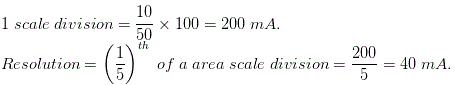# MCQs on Electrical And Electronics Measurements

##### Page 6 of 19. Go to page 1 2 3 4 5 6 7 8 9 10 11 12 13 14 15 16 17 18 19
01․ Resolution of an instrument is
the maximum non linearity.
the maximum quantity it can measure.
the minimum quantity it can measure.
ability to distinguish polarity.

Resolution of any instrument is the smallest change in the input signal (quantity under measured) which can be detected by the instrument. If the input is slowly increased from some arbitrary value it will be noticed that the output does not change at all until the increment exceeds a certain value.

02․ The measured value of a resistance is 10.25 ohm, whereas its value of 10.22 ohm. What is absolute error of the measurement?
0.01 ohm.
0.03 ohm.
15.36 ohm.
10.26 ohm.

Absolute error = measured value - true value.

03․ In measurement system, the function of the signal manipulating elements is to
to perform linear operation like addition and multiplication.
to perform non liner operation like clipping filtering, chopping and clamping.
change the magnitude of the input signal while retaining its identity.
change the quantity under measurement to an analogues signal.

Manipulation is necessary to change signal level without changing the information contained it for the acceptance of the instruments. The function of the variable manipulation unit is to change the magnitude the signal presented to it while preserving the original nature of signal.

04․ A pressure measurement instrument is calibrated between 10 bar and 260 bar. The scale span of the instrument is
10 bar.
250 bar.
260 bar.
270 bar.

The lower range of the instrument is 10 bar and upper measurement range of the instrument is 260 bar. So the scale span of the instrument is the difference between upper range and lower range measurement value instrument.

05․ If two meters X and Y require 40 mA and 50 mA respectively, to give full scale deflection, then
Y is more sensitive.
X is more sensitive.
Both X and Y are equally sensitive.
It would not be possible to assess the sensitivity on the basis of the given data.

If there are ‘S’ equal divisions in the full scale, then resolution of X and Y meters are 40/S mA and 50/S mA respectively. So the resolution of meter X is less than resolution of Y meter.

06․ Purely mechanical instrument cannot be used for dynamic measurements because they have
high inertia.
higher response time.
large time constant.
all of the above.

Mechanical instruments are unable to response rapidly to the measurement of dynamic conditions due to the fact that they have moving parts that are rigid, heavy and bulky and consequently have a large mass and they take large response time. Mass presents inertia problems and hence these instrument can not faithfully follow the rapid changes which are involved in dynamic instrument.

07․ An analog ammeter is
a recording instrument.
a controlling instrument.
an absolute instrument.
an indicating instrument.

An analog ammeter indicates the magnitude of current at the time when it is being measured. The indications are given by a pointer moving over a calibrated scale. So an analog meter is an indicating instrument.

08․ In AC circuits, the connection of measuring instruments cause loading effect errors which may effect
only the magnitude of the quantity being measured.
only phase of the quantity being measured.
both of above.
magnitude, phase and waveform of the quantity being measured.

The incapability of a system to faithfully measure, record or control the measured signal in undistorted form is known as load effect. This results in loading errors. However, under practical conditions, it has been observed that the introduction of any element of system results invariably in extraction of the energy from the system, thereby distorting the original signal. This distortion may take form of attenuation waveform distortion, phase shift, etc and consequently the ideal measurement becomes impossible.

09․ A wattmeter reads 30.34 W. The absolute error in the measurement is - 2.22 W. What is the true value of power?
32.56 W .
28.12 W.
25.45 W.
None of the above.

True value is equal to difference between measured value and absolute error.

10․ A moving coil ammeter has a uniform scale with 50 division and gives a full scale reading 10 A. The instrument can read up to (1/5)th of a scale division with a fair degree of certainty. What is the resolution of the instrument in mA?
25 mA.
40 mA.
50 mA.
80 mA.<<<45678>>>